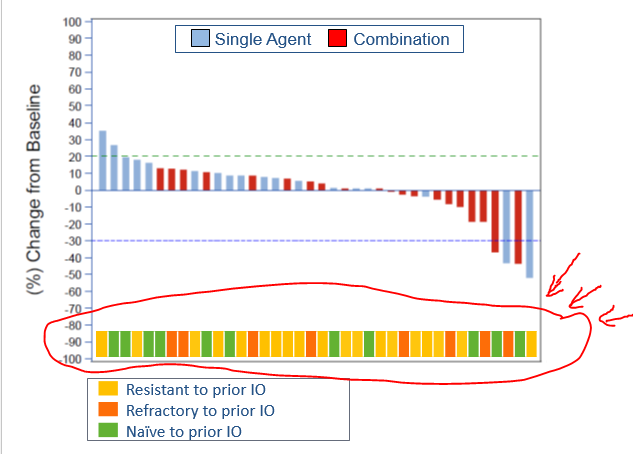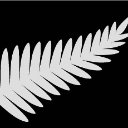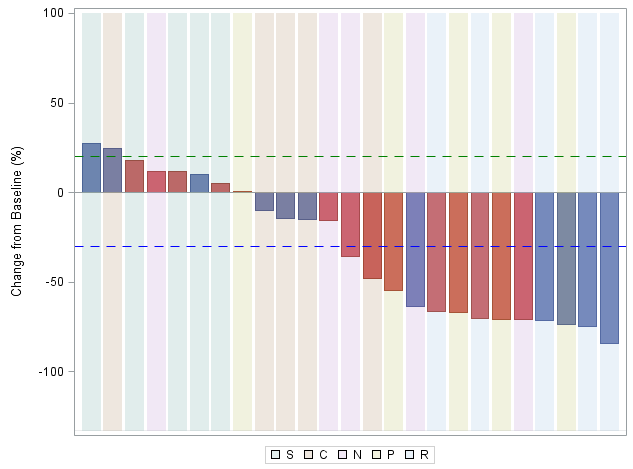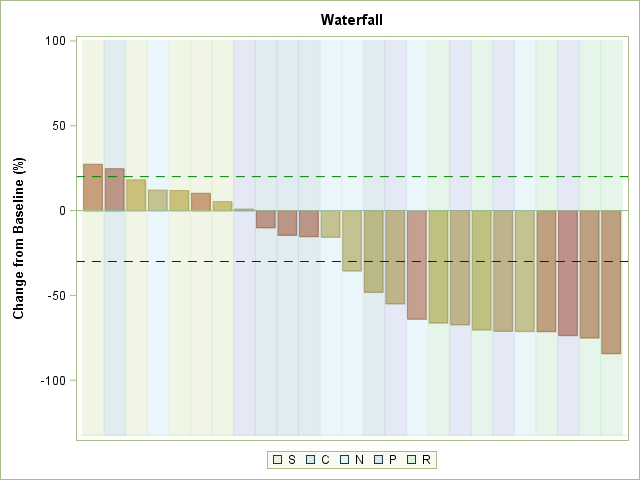## Waterfall plot

Does anyone know if the circled area in the screenshot below is possible to program?  It was manually inserted, thanks.4 REPLIES 4

## Re: Waterfall plot

It's probably possible using either POLYGON or possibly a MARKER PLOT since they're all the same size

This assumes you're using SGPLOT or SG procedures in general though.DanH_sas
SAS Super FREQ

## Re: Waterfall plot

Using data from Sanjay's post on waterfall charts (https://blogs.sas.com/content/graphicallyspeaking/2017/07/30/clinical-graphs-waterfall-plot/), I created a little example that should get you going. The key here is using the Y2AXIS and adjusting the offsets of both the YAXIS and Y2AXIS so that the overlaid bar charts do not collide. You can use an attributes map to control the colors of the "coding" bar chart.

Hope this helps!

Dan

``````data TumorSize;
length Cid \$ 3;
label Change='Change from Baseline (%)';
do Id=1 to 25;
cid=put(id, 2.0);
change=30-120*ranuni(2);
Group=ifc(int(ranuni(2)+0.5), 'Treatment 1', 'Treatment 2');
Duration=floor(50+100*ranuni(2));
Drop=ifn(ranuni(2) < 0.3, floor(duration-10), .);
if mod(id, 5) = 1 then Label='C';
if mod(id, 5) = 2 then label='R';
if mod(id, 5) = 3 then label='S';
if mod(id, 5) = 4 then label='P';
if mod(id, 5) = 0 then label='N';
output;
end;
run;

/* Add dummy response value for the "coding" bar chart */
data temp;
set TumorSize;
retain dummyvar 1;
run;

/* Sort it */
proc sort data=temp out=TumorSizeSort;
by descending change;
run;

/* Draw it */
title "Waterfall";
proc sgplot data=TumorSizeSort;
xaxis display=none discreteorder=data;
yaxis offsetmin=0.15 min=-100 max=100;
y2axis display=none offsetmax=0.9;
vbarparm category=id response=change / group=group;
vbarparm category=id response=dummyvar / group=label y2axis name="code";
refline 20 / lineattrs=(color=green pattern=dash);
refline -30 / lineattrs=(color=blue pattern=dash);
keylegend "code";
run;``````ChrisNZ
Tourmaline | Level 20

## Re: Waterfall plot

None of my business, but if the bottom bars mean what I think, the chart may be easier to read this way:( it would probably be better to remove the space between the background bars but I couldn't).

``````data TumorSize;
length Cid \$ 3;
label Change='Change from Baseline (%)';
do Id=1 to 25;
cid=put(id, 2.0);
change=30-120*ranuni(2);
Group=ifc(int(ranuni(2)+0.5), 'Treatment 1', 'Treatment 2');
Duration=floor(50+100*ranuni(2));
Drop=ifn(ranuni(2) < 0.3, floor(duration-10), .);
if mod(id, 5) = 1 then Label='C';
if mod(id, 5) = 2 then label='R';
if mod(id, 5) = 3 then label='S';
if mod(id, 5) = 4 then label='P';
if mod(id, 5) = 0 then label='N';
output;
end;
run;

/* Add dummy response value for the "coding" bar chart */
data temp;
set TumorSize;
retain dummyvar 11;
run;

/* Sort it */
proc sort data=temp out=TumorSizeSort;
by descending change;
run;

/* Draw it */
title "Waterfall";
proc sgplot data=TumorSizeSort;
xaxis display=none discreteorder=data;
yaxis offsetmin=0.15 min=-100 max=100;
y2axis display=none offsetmax=0.9 min=0 max=1;
vbarparm category=id response=change   / group=group ;
vbarparm category=id response=dummyvar / group=label y2axis name="code" transparency=.8  barwidth=1  nooutline;
refline 20 / lineattrs=(color=green pattern=dash);
refline -30 / lineattrs=(color=blue pattern=dash);
keylegend "code";
run;``````

## Re: Waterfall plot

@ChrisNZ, the inability to get the background bars without space might be choice of style and (guessing here) whether it uses a bar outline color.

I ran you code using style Meadow and get:Discussion stats
• 4 replies
• 1733 views
• 1 like
• 5 in conversation